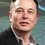The fundamental note

1) ABZP is a rectangle and ABCD and CXYZ are squares whose areas add up to 1 .Maximum possible area of the rectangle ABZP-

2) Let A , B and C be 3 circles lying in the same quadrant each touching both the axes . Suppose also that A touches B and B touches C . If the area of the smallest circle is 1 unit , then area of largest circle is -

3) Consider an equilateral triangle ABC with side 2.1 cm. You want to place a number of smaller equilateral triangles , each with side 1 cm , over the triangle ABC , so that triangle ABC is fully covered. What is the minimum number of smaller triangles needed

NCERT problems

4) $\displaystyle \int \dfrac{\sqrt{x}}{\sqrt{a^3 - x^3}} dx$

5) $\displaystyle For~ all~ g: R \to R ~ \int_{0}^{1} (t - g(t))^2 dt ~is~ positive$

6) f(x) is a non-negative continuous function such that $f(x) + f(\dfrac{1}{2} + x) = 1$ for all $x , 0 \leq x \leq \dfrac{1}{2}$ , then $\displaystyle \int_{0}^{1} f(x)dx =$Note by U Z
4 years, 8 months ago

This discussion board is a place to discuss our Daily Challenges and the math and science related to those challenges. Explanations are more than just a solution — they should explain the steps and thinking strategies that you used to obtain the solution. Comments should further the discussion of math and science.

When posting on Brilliant:

• Use the emojis to react to an explanation, whether you're congratulating a job well done , or just really confused .
• Ask specific questions about the challenge or the steps in somebody's explanation. Well-posed questions can add a lot to the discussion, but posting "I don't understand!" doesn't help anyone.
• Try to contribute something new to the discussion, whether it is an extension, generalization or other idea related to the challenge.
• Stay on topic — we're all here to learn more about math and science, not to hear about your favorite get-rich-quick scheme or current world events.

MarkdownAppears as
*italics* or _italics_ italics
**bold** or __bold__ bold

- bulleted
- list

• bulleted
• list

1. numbered
2. list

1. numbered
2. list
Note: you must add a full line of space before and after lists for them to show up correctly
paragraph 1

paragraph 2

paragraph 1

paragraph 2

> This is a quote
This is a quote
# I indented these lines
# 4 spaces, and now they show
# up as a code block.

print "hello world"
# I indented these lines
# 4 spaces, and now they show
# up as a code block.

print "hello world"
MathAppears as
Remember to wrap math in $$...$$ or $...$ to ensure proper formatting.
2 \times 3 $2 \times 3$
2^{34} $2^{34}$
a_{i-1} $a_{i-1}$
\frac{2}{3} $\frac{2}{3}$
\sqrt{2} $\sqrt{2}$
\sum_{i=1}^3 $\sum_{i=1}^3$
\sin \theta $\sin \theta$
\boxed{123} $\boxed{123}$

Sort by:

LOL ! If All These are NCERT Problem in this Year , Then I think Math will be tough for This Year CBSE Board , And Thank god I passed 12th Board earlier :) Just Joking

- 4 years, 8 months ago

Hi, in which college are you studying ?

- 4 years, 8 months ago

Hi , I'am not in collage . I'm in 13th

- 4 years, 8 months ago

@Azhaghu Roopesh M @KARAN SHEKHAWAT try atleast one question

- 4 years, 8 months ago

Seriously they are a kind of NCERT problem ( contains very basic concepts)

- 4 years, 8 months ago